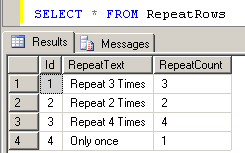﻿ Repeat Rows N Times using SQL based on a Column ValueSQL Server and T-SQL Development Tutorials Development resources, articles, tutorials, code samples, tools and downloads for ASP.Net, SQL Server, Reporting Services, T-SQL, Windows, AWS, SAP HANA and ABAP

# SQL Repeat Rows N Times According to Column Value

To repeat table rows in different number of times SQL developers can use given T-SQL codes in this SQL tutorial. Sometimes developers are required to repeat records according to a number column in that table rows.
For example, you have a master table and sql developers should create new repeating rows in an other database table for that source row. Assume that the number of rows to insert are varying for each source row according to a numeric table column in the source table. In this case given sql codes in this tutorial will help SQL Server developers coding in SQL to repeat rows.

RepeatRows is a sample database table where the source rows are stored. The requested repeating number is stored in the column named RepeatColumn in the database table RepeatRows.

You can see the database table populated with sample data in below screenshot.Now with the help of a SQL numbers table, or a SQL user function which returns a temporary numbers table I created the following SQL SELECT statement. Please note the use of CROSS APPLY operator which joins the RepeatRows table and data returning from SQL numbers table function NumbersTable. The trick in this SQL code is passing the RepeatCount column value of the source table to the SQL function NumbersTable. And this SQL Select will repeat rows according to the count column in the same table record.

Please refer to Create SQL numbers table in SQL Server for the source codes of the user function dbo.NumbersTable used in this Transact-SQL tutorial.

The output of the above SQL statement is as follows. As you see each source row is repeated n times (according to the RepeatCount column value of the source row) in the return set of the SELECT statement.An other repeating records sample case can be like this. Assume that we have a computer cource class where students are taking exams. The number of the students taking course exams are stored in database table named Exams. And we will store exam scores in target database table ExamScores.

Here is the DDL codes for the sql tables and script for data generation. We want to repeat rows stored in this table n times defined in the NumberOfStudent numeric column value.

Now we have two exam records in our source table. Let's insert new rows for the exam scores for the exam candidates in ExamScores table by repeating the rows as the number of exam takers in source table.

Again for multiplying records and repeating them for a specific number of times, I'll use the SQL SELECT statement in combination with NumbersTable sql function and CROSS APPLY operator. Here is the DDL source code for ExamScores table.

And the below SQL codes repeat source row with the number of times defined in a column of that table row.

I hope this SQL tutorial helps SQL programmers repeat a table row n times using SQL Server Numbers table and Cross Apply join. I believe this tutorial will give an idea how to use Cross Apply in their SQL development for data professionals

SQL Server